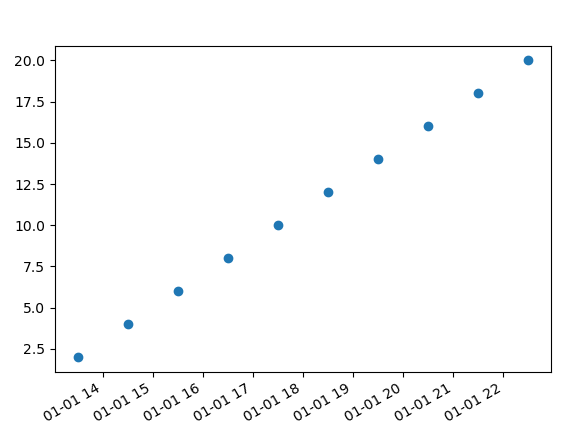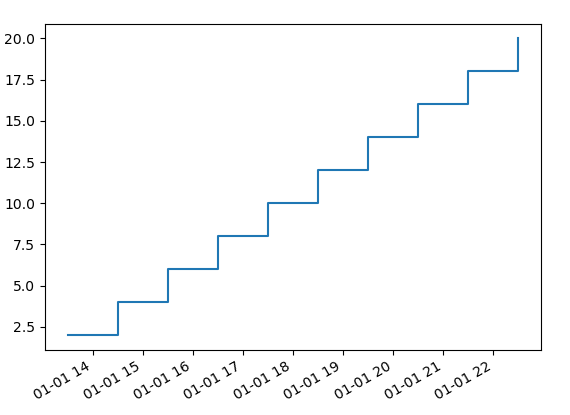# Plot_date not applying drawstyle='steps-post'

I tried the following code -
import matplotlib

import matplotlib.pyplot as plt

import datetime

customdate = datetime.datetime(2016, 1, 1, 13, 30)

y = [ 2,4,6,8,10,12,14,16,18,20 ]

x = [customdate + datetime.timedelta(hours=i) for i in range(len(y))]

_fig,_ax = plt.subplots()

# plot

_ax.plot_date(x,y, drawstyle=‘steps-post’)

plt.gcf().autofmt_xdate()

plt.show()

and got the output as -The step plot is not created, but when I change to